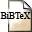# On the capacity of UMTS with directional antennas

## Abstract

Forthcoming UMTS networks in Europe will be based on code divison multiple access with a constant chip rate of 3.84 MChips/s. After despreading and filtering the bit energy-to-noise ratio is given by the product of the spreading gain times the actual carrier-to-interference ratio. Power control applies such that each mobile uses the minimum transmission power to achieve a bit energy-to-noise ratio of 5 dB. The interactions between the above quantities can be described by a system of linear equations, whose solution gives insight into the maximum capacity of a cell. This allows for dealing with the probability of exceeding the maximum possible traffic rate of a cell. Assuming a stochastic user model with random locations and random data rates, the corresponding outage probability is determined for omnidirectional and beam forming antennas.

## BibTEX Reference Entry```@inproceedings{MaRe01,
author = {Rudolf Mathar and Marie-Ange Remiche},
title = "On the capacity of {UMTS} with directional antennas",
pages = "71-75",
booktitle = "10th Aachen Symposium on Signal Theory",
year = 2001,
hsb = RWTH-CONV-223538,
}
```Download bibtex-file